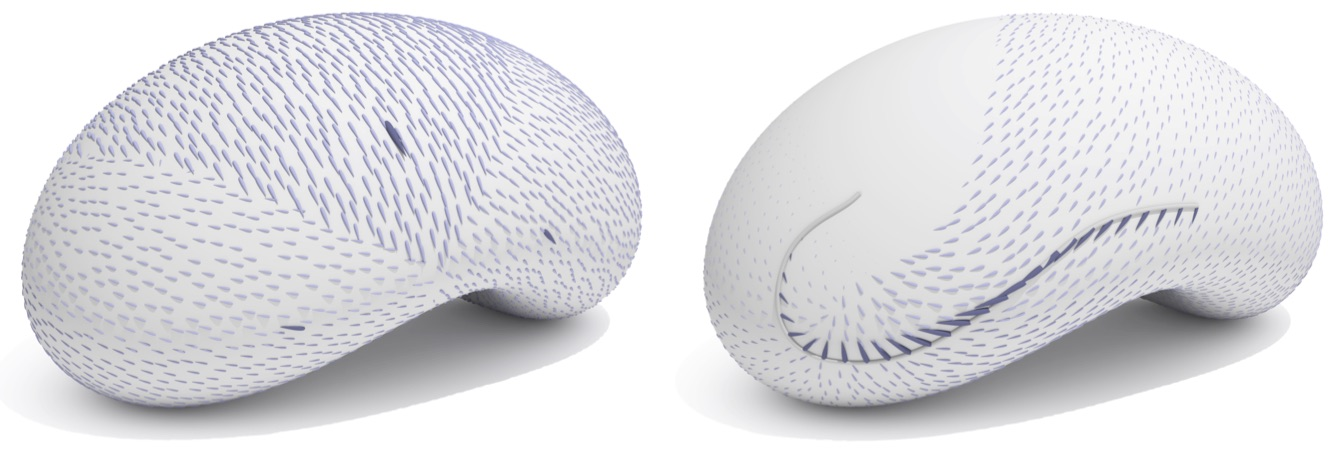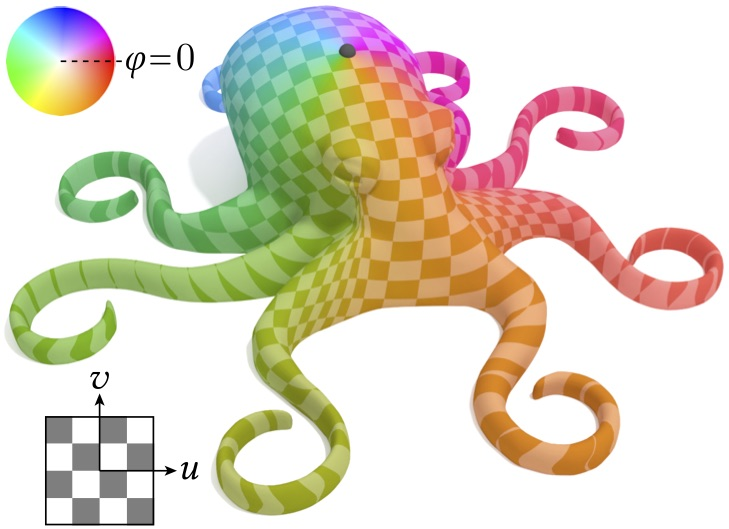# Vector Heat Method

This section describes the Vector Heat Method in geometry-central, which computes parallel transport of vectors using heat flow, and applications that follow from it.

Note that these quantities all depend on the intrinsic geometry of a surface (via the IntrinsicGeometryInterface). Therefore, these routines can be run on abstract geometric domains as well as traditional surfaces in 3D.

These algorithms are described in The Vector Heat Method.

#include "geometrycentral/surface/vector_heat_method.h"

## Vector Heat Solver

The stateful class VectorHeatSolver shares precomputation for all of the routines below.

VectorHeatSolver::VectorHeatSolver(IntrinsicGeometryInterface& geom, double tCoef=1.0)

Create a new solver for the Vector Heat Method. Precomputation is perfrmed lazily as needed.

• geom is the geometry (and hence mesh) on which to compute. Note that nearly any geometry object (VertexPositionGeometry, etc) can be passed here.

• tCoef is the time to use for short time heat flow, as a factor m * h^2, where h is the mean edge length. The default value of 1.0 is almost always sufficient.

Algorithm options (like tCoef) cannot be changed after construction; create a new solver object with the new settings.

## Scalar Extension

Given scalar data defined at isolated source locations on a surface, extend it to the entire domain. Each point on the domain will take the value of the nearest source point.Example:

// your mesh and geometry
VertexPositionGeometry geometry;
HalfedgeMesh mesh;

// construct a solver
VectorHeatSolver vhmSolver(geometry);

// some interesting source values
std::vector<std::tuple<Vertex, double>> points;
for (/* ... some inputs ... */ ) {
Vertex sourceVert = /* something */;
double sourceVal = /* something */;
points.emplace_back(sourceVert, sourceVal);
}

// solve!
VertexData<double> scalarExtension = vhmSolver->extendScalar(points);


VertexData<double> VectorHeatSolver::extendScalar( const std::vector<std::tuple<Vertex, double>>& sources)

Compute the nearest-neighbor extension of scalar data defined at isolated vertices to the entire domain. The input is a list of vertices and their corresponding values.

VertexData<double> VectorHeatSolver::extendScalar( const std::vector<std::tuple<SurfacePoint, double>>& sources)

Compute the nearest-neighbor extension of scalar data defined at isolated points to the entire domain. The input is a list of surface points and their corresponding values.

## Vector ExtensionGiven tangent vectors defined at one or more isolated source locations on a surface, extend transport the vectors across the entire domain according to parallel transport. Each point on the domain will take the value of the nearest source point.

VertexData<Vector2> VectorHeatSolver::transportTangentVectors(Vertex sourceVert, Vector2 sourceVec)

Compute the parallel transport of a vector defined at a single vertex to the entire domain. The input is defined in the tangent space of the source vertex.

VertexData<Vector2> VectorHeatSolver::transportTangentVectors( const std::vector<std::tuple<Vertex, Vector2>>& sources)

Compute the parallel transport of vectors defined at a collection of vertices to the entire domain. The input is defined in the tangent space of each the source vertex.

VertexData<Vector2> VectorHeatSolver::transportTangentVectors( const std::vector<std::tuple<SurfacePoint, Vector2>>& sources)

Compute the parallel transport of vectors defined at a collection of surface points to the entire domain. The input is defined in the tangent space of each the vertex, face, or edge respectively.

## Logarithmic Map

The logarithmic map is a very special 2D local parameterization of a surface about a point, where for each point on the surface the magnitude of the log map gives the geodesic distance from the source, and the polar coordinate of the log map gives the direction at which a geodesic must leave the source to arrive at the point.These routines compute the logarithmic map using the vector heat method.

VertexData<Vector2> VectorHeatSolver::computeLogMap(const Vertex& sourceVert)

Compute the logarithmic map with respect to the given source vertex.

The angular coordinate of the log map will be respect to the tangent space of the source vertex.

VertexData<Vector2> VectorHeatSolver::computeLogMap(const SurfacePoint& sourceP)

Compute the logarithmic map with respect to the given source point, which is a general surface point.

The angular coordinate of the log map will be respect to the tangent space of the source vertex, edge, or face.

## Citation

If these algorithms contribute to academic work, please cite the following paper:

@article{sharp2019vector,
title={The Vector Heat Method},
author={Sharp, Nicholas and Soliman, Yousuf and Crane, Keenan},
journal={ACM Transactions on Graphics (TOG)},
volume={38},
number={3},
pages={24},
year={2019},
publisher={ACM}
}